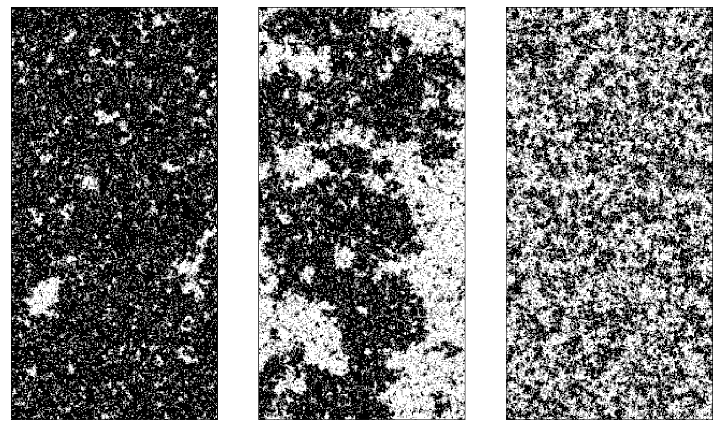The Spin-1 Blume-Capel model is a generalization of the standard Ising model with dynamics described by the Hamiltonian

$\mathcal{H} = J\sum\limits_{\langle i,j\rangle} \phi_i\phi_j + D\sum\limits_{i} \phi_i^2,$

where the spins take on integer values $$\phi_i=-1,0,+1$$. Despite its relative simplicity, the Blume-Capel model features a rich physical phenomenology, most notably a second-order transition line, which becomes first-order for low temperatures. The second term in the Hamiltonian gives rise to a zero-field splitting, raising the energy of the $$\phi=\pm 1$$ states above the $$\phi=0$$ state. The parameter $$D$$ is commonly referred to as the crystal-field coupling. Although originally proposed in the context of magnetic materials12, the model can also be applied to, e.g., $$^3 \text{He}$$ - $$^4 \text{He}$$ mixtures3, where the quadrupole moment $$1-\langle \phi^2\rangle$$ corresponds to the fractional $$^3\text{He}$$-concentration.Figure 1. Two-dimensional Blume-Capel model slightly below (left), at (middle), and slightly above (right) the critical transition point. Spin configurations $$\phi=\pm1$$ are displayed in black/white colors, whereas the neutral state $$\phi=0$$ is shown in gray.

In Figure 1, we present typical configurations of the ferromagnetic Blume-Capel model, below, at, and above the critical temperature, for $$D=1$$ fixed. Only in the panel showing the critical configuration one finds a fractional appearance, indicating the lack of scales which is characteristic at a continuous transitions. In the left panel, one magnetic orientation dominates, as the bimodal symmetry is spontaneously broken. The right panel, in contrast, features relatively featureless high temperature disorder and hence no clusters of significant spatial extension.

For simulating the model we use a hybrid algorithm, combining Wolff cluster updates and local Metropolis steps. This way the update scheme remains ergodic, as the cluster update only builds cluster on the either of the $$\phi=\pm 1$$ spins, leaving the $$\phi=0$$ untouched.

1. Blume, M. (1966). Theory of the First-Order Magnetic Phase Change in UO$$_2$$. Physical Review, 141(2), 517.
2. Capel, H. W. "On the possibility of first-order phase transitions in Ising systems of triplet ions with zero-field splitting." Physica 32.5 (1966): 966-988.
3. Blume, M., Emery, V. J., & Griffiths, R. B. (1971). Ising model for the $$\lambda$$ transition and phase separation in He3-He4 mixtures. Physical review A, 4(3), 1071.